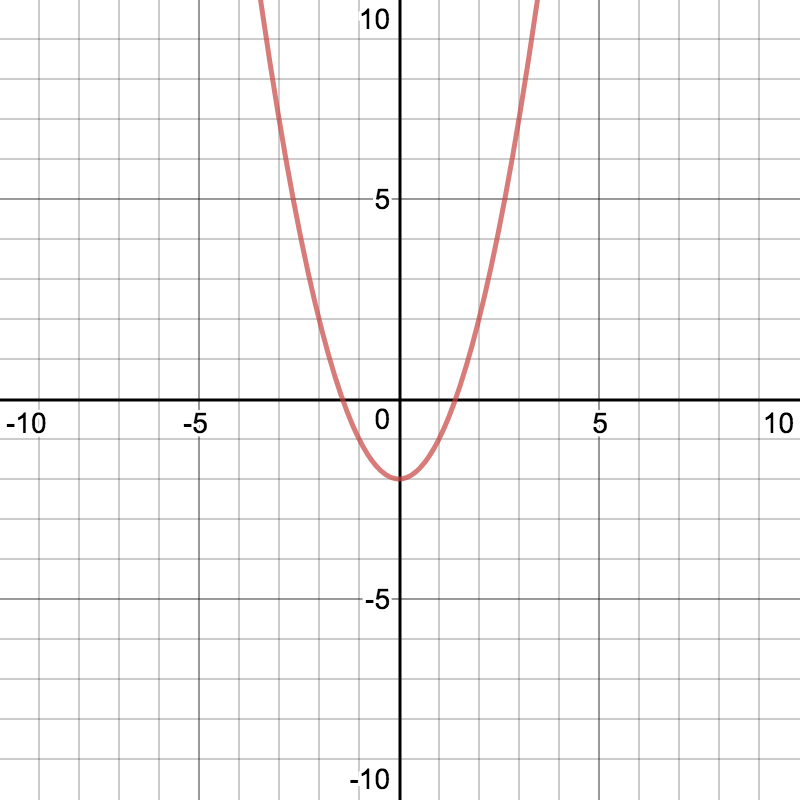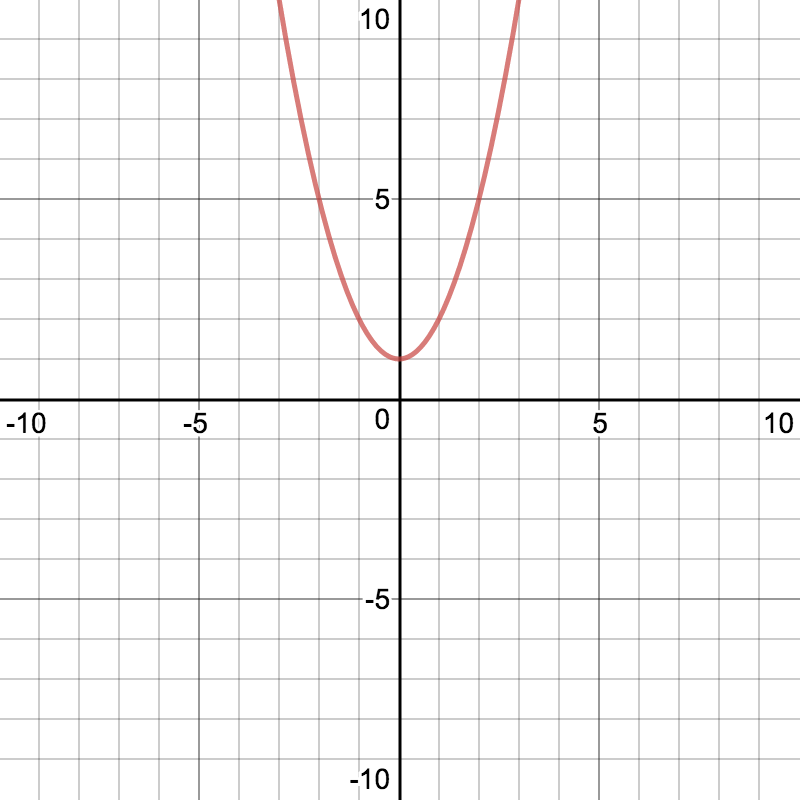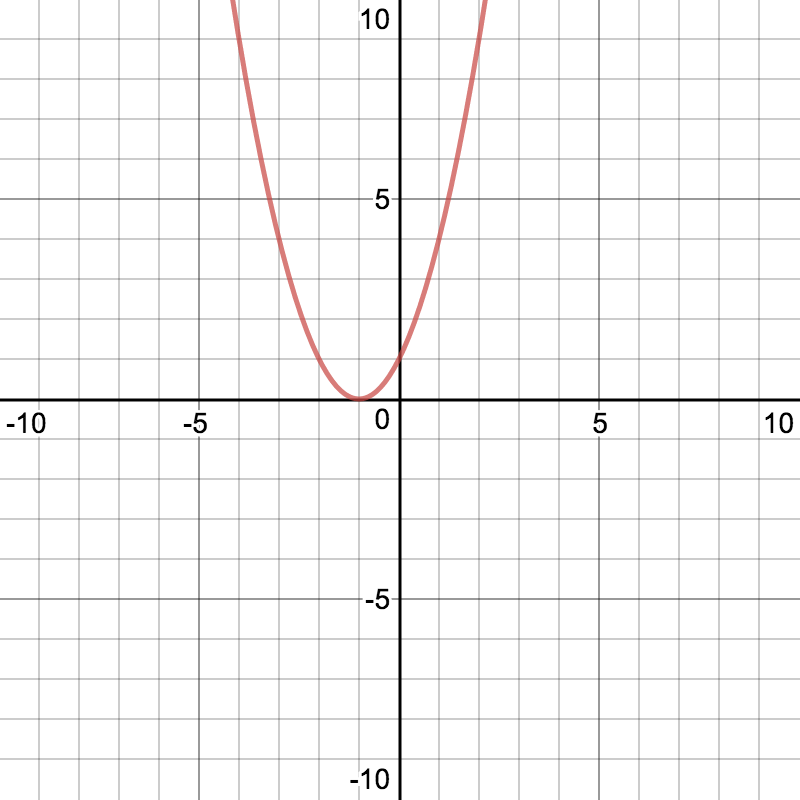# FNGR 2 | Lesson 4 | PracticeDescribe the transformation of the parent function, $$f(x) = x^2$$. Match the graph to the algebraic representation. Graphs are created using Desmos.com.

 1.2.3.4.5.6.a) $$f(x) = x^2+1$$

b) $$f(x) = x^2 -2$$

c) $$f(x) = (x+1)^2$$

d) $$f(x) = (x-5)^2$$

e) $$f(x) = (x+2)^2 -2$$

f) $$f(x) = -2(x-1)^2+3$$